# WBJEE 2020 Chemistry Paper with Solutions

Students will find WBJEE 2020 Chemistry question paper along with solutions on this page. Our specialized experts have prepared a detailed and step by step solutions for all the questions asked in the exam paper. These solutions will help students to understand the problem and its correct answer. More significantly, students can use the paper to study effectively and solving the paper will also further help them develop adept speed and accuracy to tackle all the problems in the upcoming exam. Students can easily download the WBJEE question paper PDF for offline use.

### WBJEE 2020 - Chemistry

Question 1. What will be the mass of one atom of 12C:

1. a. 1 amu
2. b. 1.9923×10–23g
3. c. 1.6603 × 10–22 g
4. d. 6 amu

Solution:

Mole concept

1 mole of element = atomic mass of atom

1 mole of element = 6.022 × 1023 atoms

So, mass of one mole 12C = 12g

One mole of 12C contains = 6.022 × 1023 atoms

Mass of 1 atom of 12C = 12g ×1 12C atom/6.022 × 102312C atom

= 1.9923 × 10–23g

Question 2. The bond order of He2, He2+ and He22+ are respectively.

1. a. 1, ½, 0
2. b. 0, ½, 1
3. c. ½, 1, 0
4. d. 1, 0, ½

Solution:

Bond order = (NBMO - NABMO)/2

Where NBMO = number of Bonding molecular orbital's electrons

NABMO = number of anti bonding molecular orbital's electrons.

Electronic configuration of He2 = (σ1s)2 (σ*1s)2

From the above electronic configuration,

Bond order = (Nb-Na)/2

= (2-2)/2

= 0

Same as for other molecules

Electronic configuration of He2+: (σ1s)2 (σ*1s)1

Bond order = (2-1)/2

= 1/2

Electronic configuration of He2+2: (σ1s)2 (σ*1s)0

Bond order = (2-0)/2 = 1

So, Bond order of He2, He2+ and He22+ are respectively 0, 1/2 , 1

Question 3. To a solution of a colourless efflorescent sodium salt, when dilute acid is added, a colourless gas is evolved along with formation of a white precipitate. Acidified dichromate solution turns green when the colourless gas is passed through it. The sodium salt is

1. a. Na2SO3
2. b. Na2S
3. c. Na2S2O3
4. d. Na2S4O6

Solution:

When the salt reacts dilute acid, sulphur dioxide gas is liberated.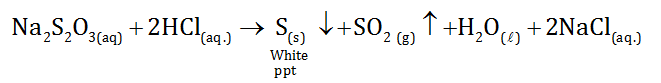Na2SO3 and Na2S4O6 both produce SO2 on treatment with HCl but does not give white precipitate of sulphur.

Acidified dichromate solution turns green on passing SO2 gas.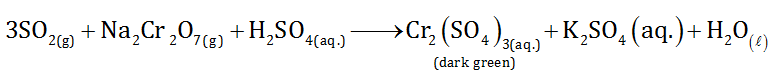Question 4. The reaction for obtaining the metal (m) from its oxide M2O3 ore is given by

M2O3(s) + 2Al(l) Al2O3(l) + 2M(s),(s = solid, l = liquid) in the case, M is

1. a. Copper
2. b. Calcium
3. c. Iron
4. d. Zinc

Solution:

Thermite reaction: A thermite reaction is demonstrated by igniting a mixture of aluminium and iron oxide generating molten iron and aluminium oxide.

This reaction is an oxidation-reduction reaction, a single replacement reaction.

Question 5. In the extraction of Ca by electro reduction of molten CaCl2 some CaF2 is added to the electrolyte for the following reason :

1. a. To keep the electrolyte in liquid state at temperature lower than the melting point of CaCl2
2. b. To effect precipitation of Ca
3. c. To effect the electrolysis at lower voltage
4. d. To increase the current efficiency

Solution:

In the extraction of calcium by electro-reduction of molten Calcium chloride (CaCl2), some amount of CaF2 is added to keep the electrolyte in liquid state at temperature lower than the melting point of CaCl2. As it is known, the electrolyte can be decomposed if the potential applied is high enough.

Question 6. The product in the below reaction is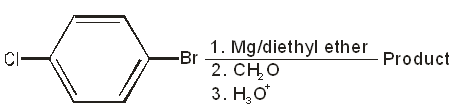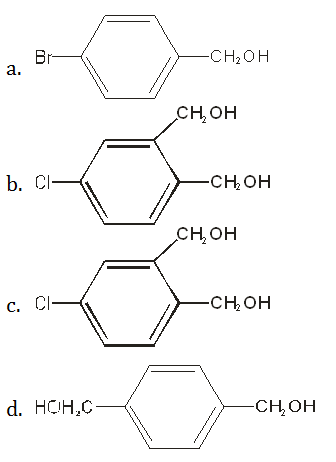Solution: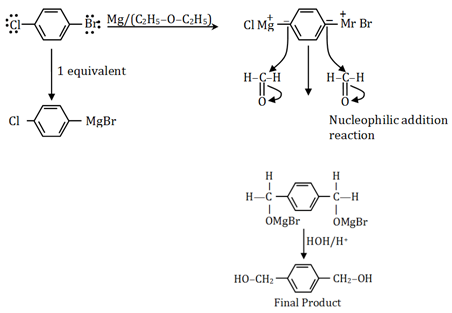Question 7. For the below three esters, the order of rates of alkaline hydrolysis is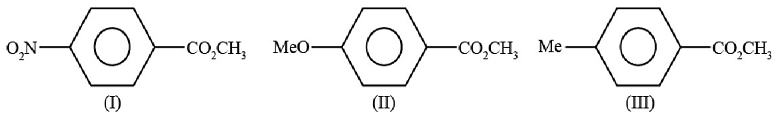1. a. I > II > III
2. b. II > III > I
3. c. I > III > II
4. d. III > I > II

Solution: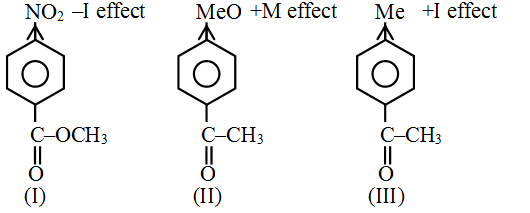Under alkaline condition, hydroxide attacks on carbonyl carbon of esters to furnish respective acids.

So, when the partial positive charge on carbonyl carbon increases, attack of OH becomes more favorable and rate of hydrolysis increases.

– I effect ∝ 1/positive charge

+M effect ∝ positive charge

+I effect ∝ positive charge

+M effect is dominant than +I effect.

Hence, order of rate of alkaline hydrolysis I > III > II.

Question 8. This alcohol is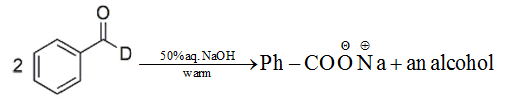1. a. Ph-CHD-OH
2. b. Ph-CHD-OD
3. c. Ph-CD2-OH
4. d. Ph-CD2-OD

Solution:

Cannizaro reaction is a redox reaction in which two molecules of aldehyde react to give oxidation product which is a salt of a carboxylic acid and the reduction product which is an alcohol, under basic condition.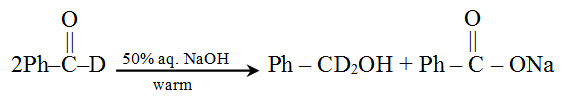Question 9. The correct order of acidity for the following compound is :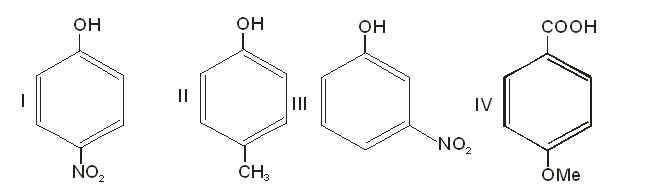1. a. II < IV < III < I
2. b. II < III < I < IV
3. c. II < III < IV < I
4. d. III < II < I < IV

Solution:

Acidity ∝ resonance

Acidity ∝ –I effect

Anything which stabilizes the conjugate base will increase the acidity. Resonance increases the stability of conjugate base because the negative charge can be delocalized i.e. COO group.

Electron withdrawing substituent’s (–NO2 group) can increase acidity of a nearby atom.

–NO2 group is ortho/para directing group that shows maximum acidity at para position.

CH3 group shows +I effect which destabilize conjugate base.

So, compound I is more acidic than compound II.

Hence, the acidity order is II < III < I < IV.

Question 10. For the following carbo cations, the correct order of stability is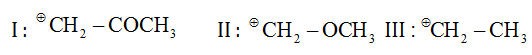1. a. III < II < I
2. b. II < I < III
3. c. I < II < III
4. d. I < III < II

Solution:

Stability of carbocations depends on following factors:

Stability of carbocation ∝ + M or +I effect

Stability of carbocation ∝ Hyper conjugation

Stability of carbocation ∝ 1/-I effect.

Question 11. The reduction product of ethyl-3-oxobutanoate by NaBH4 in methanol is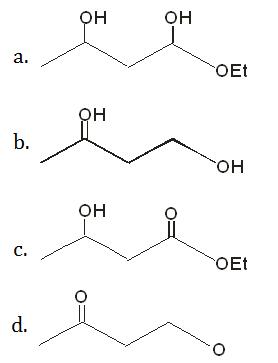Solution:

NaBH4 is the most selective reducing agent which only reacts with aldehydes or ketones due to its milder nature. So, keto group of Ethyl-3-oxobutanoate reduce only not ester group.

The reaction is as follows: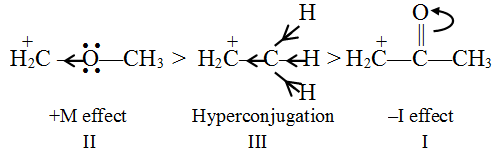Question 12. What is the major product of the following reaction?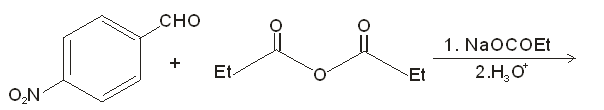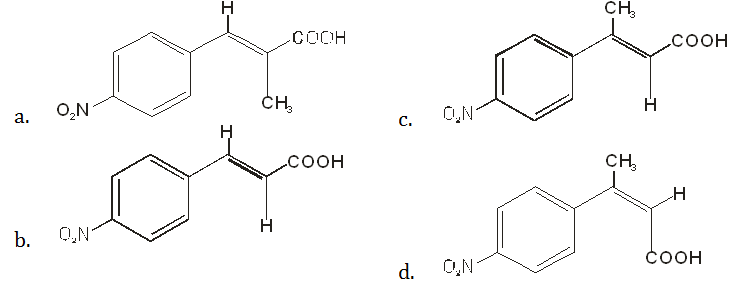Solution:

Perkin reaction is used to convert an aromatic aldehyde and an anhydride to an α, β-unsaturated carboxylic acid using sodium acetate, and acid workup.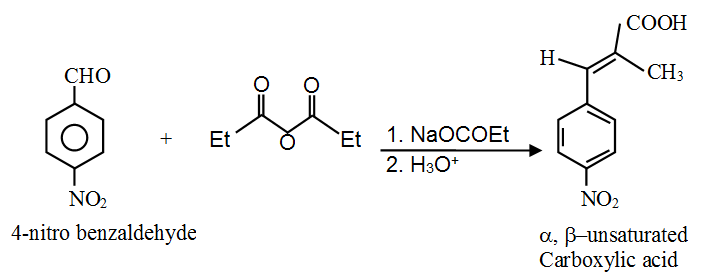Question 13. The maximum number of electrons in an atom in which the last electron filled has the quantum number n = 3, I = 2 and m = -1 is

1. a. 17
2. b. 27
3. c. 28
4. d. 30

Solution:

Principle Quantum number (n) = 3 shell

Azimuthal Quantum number (l) = 2

l = 0, 1, 2

s, p, d

It means last electron enters in 3d sub shell

Value of magnetic Quantum number (m) = -1

We know, m can be +2, +1, 0, -1,-2.

While filling electron's in d-orbital, we can find out values of m for the last electron.

For atomic number 27, values of m can be +1/-1.

For atomic number 28, the value of m is 0.

For atomic number 30, values of m can be +2/-2.

So, by analyzing the data we can say that for atomic number 27 only m can be +1/-1.

So, electronic configuration is 1s22s22p63s23p614s23d7.

Maximum number of electrons in Co: 27

Question 14. In the face-centred cubic lattice structure of gold, the closest distance between gold atoms is : (‘a’ being the edge length of the cubic unit cell)

1. a. a√2
2. b. a/√2
3. c. a/2√2
4. d. 2√2

Solution:

In FCC lattice, the closest distance is 2r.

Edge length of the cubic unit cell = a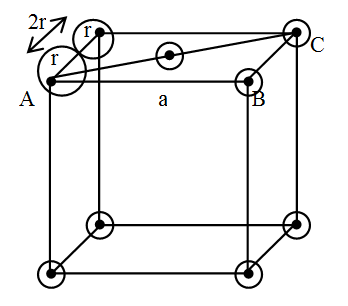In ∆ABC

According to Pythagoras theorem:

AC2 = AB2 + BC2

= a2 + a2

AC2 = 2a2

AC = √2a

d= AC/2

= √2a/2

Since d = a/√2

r (radius) = d/2 = a/2√2

2r = a/√2

In FCC closest distance between gold atoms is a/√2.

Question 15. The equilibrium constant for the following reaction are given at 250C

2A ⇌ B+C, K1 = 1.0

2B ⇌ C+D, K2 = 16

2C+D ⇌ 2P, K3 = 25

The equilibrium constant for the reaction P ⇌ A+1/2 B at 250C is 1.0

1. a. 1/20
2. b. 20
3. c. 1/42
4. d. 21

Solution:

According to the question,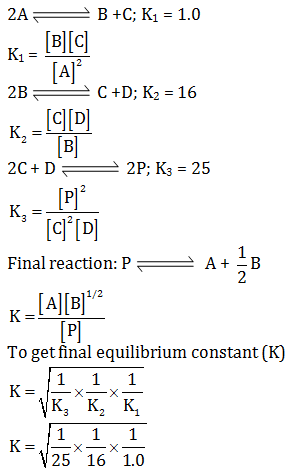K = 1/20

Question 16. Among the following, the ion which will be more effective for flocculation of Fe(OH)3 sol is:

1. a. PO43-
2. b. SO42-
3. c. SO32-
4. d. NO32-

Solution:

"According to Hardy Schulze rule, greater the valency of the active ion or flocculating ion, greater will be its coagulating power." Thus effective flocculation of Fe(OH)3 sol is:

PO43- > SO42- ≈ SO32- > NO3-

Question 17. The mole fraction of ethanol in water is 0.08. Its molality is :

1. a. 6.32 molkg–1
2. b. 4.83 molkg–1
3. c. 3.82 molkg–1
4. d. 2.84 molkg–1

Solution:

As we know,

Mole fraction of ethanol + mole fraction of water = 1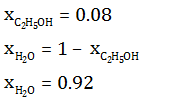Mass of water = moles × molar mass

= 0.92×18

= 16.56 g

= 0.01656 kg

Molality = moles of solute/mass of solvent (kg)

m = 0.08/0.01656

m = 4.83 mol/kg

molality of the solution is 4.83 mol/kg.

Question 18. 5 ml of 0.1 M Pb(NO3)2 is mixed with 10 ml of 0.02 M K I. The amount of PbI2 precipitated will be about

1. a. 10–2 mol
2. b. 10–4 mol
3. c. 2 × 10–4 mol
4. d. 10–3 mol

Solution:

Given,

Volume of Pb(NO3)2 = 5 ml

Concentration [Pb(NO3)2] = 0.1 M

Moles of Pb (NO3)2 = C(Concentration) × V(Volume)

= 50 x 1/1000

= 5 x 10-4 mol

Volume of KI = 10 ml

Concentration of KI = 0.02 M

Moles of KI = Concentration × volume

= 0.0210/1000

= 2×10–4mol

Pb(NO3)2+2KI -> PbI2+2KNO3

Initial moles of Pb(NO3)2 and 2KI are 5 × 10–4 mol and 2 × 10–4 mol.

so, 10–4 Limiting reagent

L.R. --> Moles of reactant is divided by its stoichiometry coefficient. Lesser moles of reactant is limiting reagent.

Because of KI being a limiting reagent, the amount of PbI2 precipitated will be 10–4 mol.

Question 19. At 273 K temperature and 76 cm Hg pressure, the density of a gas is 1.964 gL–1. The gas is

1. a. CH4
2. b. CO
3. c. He
4. d. CO2

Solution:

Given,

Density (d) = 1.964 g/L

Pressure (P) = 76 cm Hg= 760 mm Hg or 1 atm

Temperature (T) = 273 K

R = 0.0821 atm/k mol

According to ideal gas equation:

PV = nRT

PV = mR/MwtT

n = mass 9m)/Molecular weight(Mwt)

Molecular weight = mR/PVT

Density = mass (m)/Volume (V)

Mwt = dR/PT

= 1.9640 x 0821/1273

= 44g

44 g is the molecular weight of CO2 gas.

Question 20. Equal masses of ethane and hydrogen are mixed in an empty container at 298K. The fraction of total pressure exerted by hydrogen is

1. a. 15 : 16
2. b. 1 : 1
3. c. 1 : 4
4. d. 1 : 6

Solution:

According to Raoult's law, the partial vapour pressure of each volatile component in the solution is directly proportional to its mole fraction.

PH2 ∝ XH2

Suppose xg of both are mixed.

Moles of H2 = weight/molecular weight = x/2

Moles of C2H5 = x/30

Mole fraction of H2 = (x/2)/[(x/2)+(x/30)]

= (x/2)/(16x/30)

= 15/16

So, fraction of total pressure exerted by hydrogen is 15/16.

Question 21. An ideal gas expands adiabatically against vacuum. Which of the following is correct for the given process?

1. a. ∆S = 0
2. b. ∆T = - ve
3. c. ∆U = 0
4. d. ∆P = 0

Solution:

For an ideal gas expanding adiabatically,

∆U = w

Hence ∆q = 0

So, W = -Pext∆V = 0

Expansion against Pext = 0

∆U = q+W

∆U = 0

Question 22. Kf (water) = 1.86 K kg mol–1. The temperature at which ice begins to separate from a mixture of 10 mass % ethylene glycol is

1. a. -1.86 0C
2. b. -3.720C
3. c. -3.30C
4. d. -30C

Solution:

As we know

∆Tf = KfWB1000/MBWA

Given that

Kf = 1.86k kg/mol

10 mass% ⇒10g ethylene glycol mixed with 90g ice ⇒ 100 g solution

wA = iceCH2O = 90g

wB = ethylene glycol = 10g

Molecular weight of ethylene glycol (mB) = 62

So, ∆Tf = 1.8 x 6 x 10 x 1000/62 x 90

∆Tf = 3.330C

∆Tf = Tf0-Tf

Tf = ∆Tf-Tf0

Tf = -3.30C

Question 23. The radius of the first Bohr orbit of a hydrogen atom is 0.53 × 10–8 cm. The velocity of the electron in the first Bohr orbit is:

1. a. 2.188×108 cms–1
2. b. 4.376×108 cms–1
3. c. 1.094×108 cms–1
4. d. 2.188×109 cms–1

Solution:

Velocity of electron in first Bohr orbit of hydrogen atom

mvr = nh/2π

r = 0.53×10–8 m

n = 1

h = 6.626×10–26 m

m = 9.1×10–31 kg

v = nh/2πmr

On substituting the values velocity (v) = 1×6.26×10-26/(2×3.14×9.1×10-31×0.53×10-8)

= 2.18×106 m/s

= 2.18×108 cm/s

Question 24. Which of the following statement is not true for the reaction :

2F2 + 2H2O → 4HF + O2?

1. a. F2 is more strongly oxidising than O2
2. b. F–F bond is weaker than O=O bond
3. c. H–F bond is stronger than H–O bond
4. d. F is less electronegative than O

Solution:

Oxygen is less electronegative element than the fluorine having electronegativity values 3.44 and 3.98 respectively.

Hence, option D is incorrect.

Question 25. The number of unpaired electrons in the uranium (92U) atom is :

1. a. 4
2. b. 6
3. c. 3
4. d. 1

Solution:

General electronic configuration of Uranium (92U) = [Rn]5f3 6d1 7s2

Orbital diagram of filling electrons:

Filling of electrons -According to Hund’s rule -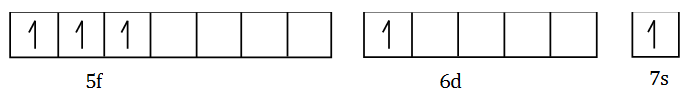So, the number of total unpaired electrons in uranium atom = 3 + 1 = 4

Question 26. The difference between the orbital angular momentum of an electron in a 4f orbital and another electron in a 4s orbitals is:

1. a. 2√3
2. b. 3√2
3. c. √3
4. d. 2

Solution:

Expression for thr orbital angular momentum

μl = √(l (l +1))h/2 π

For 4f electron, l = 3 and

For 4s electron, l = 0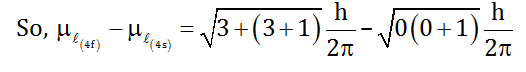= √12

= 2 √3

Question 27. Which of the following has the largest number of atoms?

1. a. 1 g of Ag
2. b. 1g of Fe
3. c. 1g of Cl2
4. d. 1g of Mg

Solution:

(A) 1g of Ag = (1/atomic weight) mole atom of Au

= (1/107)NA

= (1/107)6.022 x 1023 atoms of Au

Similarly

(B) 1g of Fe = (1/56) mole atom of Fe

= (1/56)6.0221023 atoms of Fe

(C) 1g of Cl2 = (1/71) mole atom of Cl2

= (2/71) x 6.022 x 1023 atoms of Cl2

(D) 1g of Mg = (1/24) mole atom of Mg

= (1/24)6.022 x 1023 atoms of Mg

So, 1g magnesium has the largest number of atoms.

Question 28. Indicate the correct IUPAC name of the co-ordination compound shown in the figure.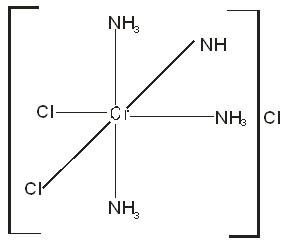1. a. Cis-dichlorotetraminochromium (III) chloride
2. b. Trans-dichlorotetraminochromium (III) chloride
3. c. Trans-tetraminedichlorochromium (III) chloride
4. d. Cis-tetraamminedichlorochromium (III) chloride

Solution: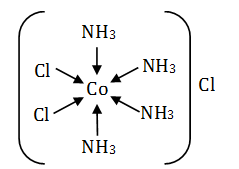IUPAC name of Co-ordination compound is: Cis-tetraminedichlorochromium (III) chloride.

To write the correct IUPAC name of the compound first we write cationic part and then anionic part. The prefix ‘tetra’ indicates the presence of four NH3 ligands prefix ‘di’ indicates two chlorine ligand.

The name of ligands is written in alphabetical order because both the ammonia and the chlorine atoms are on the same sides hence we use cis before the name of the compound.

Question 29. A homonuclear diatomic gas molecule shows 2 electrons magnetic moment. The-electron and two-electron reduced species obtained from the above gas molecule can act as both oxidizing and reducing agent. When the gas molecule is one-electron oxidized the bond length decreases compared to the neutral molecule. The gas molecule is :

1. a. N2
2. b. Cl2
3. c. O2
4. d. B2

Solution:

The gas molecule must be O2 because having two unpaired electrons in its outermost electronic configuration i.e.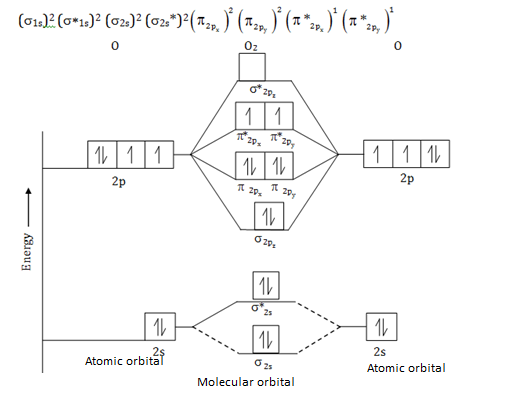After removal of one electron (oxidized), bond length decreases as compared to the neutral molecule.

O2 behaves as oxidizing as well as reducing agent by either losing or gaining of an electron.

Question 30. Which information regarding this reaction is applicable?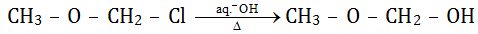1. a. It follows SN2 pathway because it is a primary alkyl chloride
2. b. It follows the SN1 pathway because the intermediate carbocation is resonance stabilized
3. c. SN1 pathway is not followed, because the intermediate carbocation is destabilized by –I effect of oxygen.
4. d. A mixed SN1and SN2 pathway is followed.

Solution: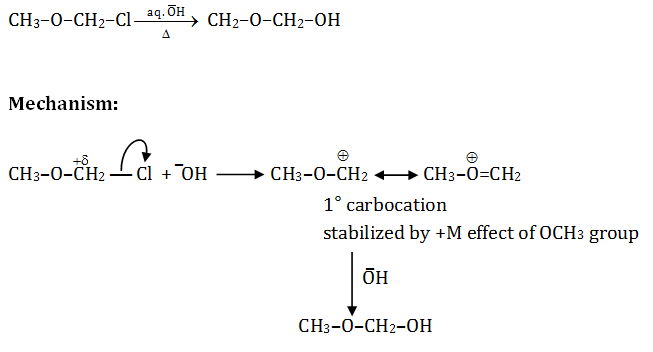It is the nucleophilic substitution reaction and follows the SN1 mechanism due to 10 stable carbocation.

SN2 is also facilitated by -OH due to response stabilization of transition state.

Question 31. Which of the following compound is asymmetric?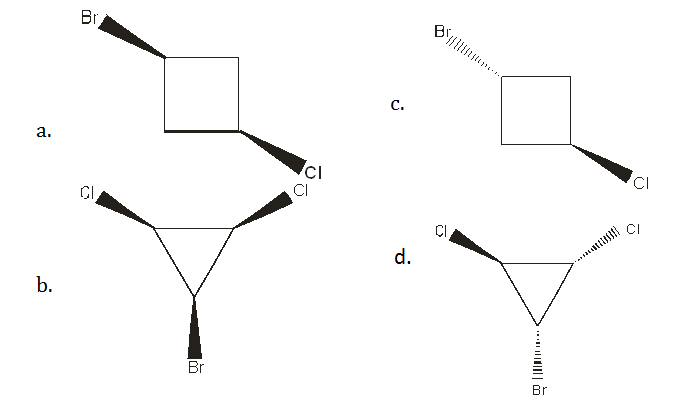Solution: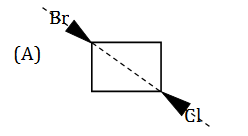Plane of symmetry is present; hence it is a symmetric molecule.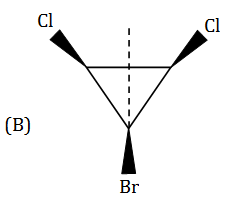Plane of symmetry is present; hence it is a symmetric molecule.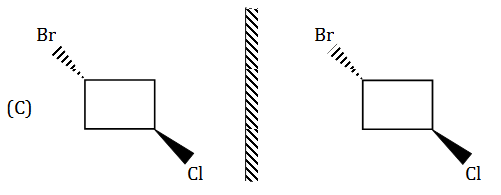Super imposable images.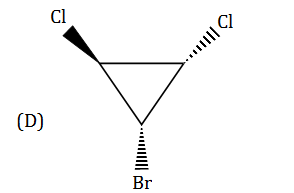Plane of symmetry absent has non-superimposable mirror image (Diastereomer). Hence it is an asymmetric compound.

Question 32. For a reaction 2A + B -> P, when the concentration of B alone is doubled, t1/2 does not change and when concentrations of both A and B is doubled, rate increases by a factor of 4. The unit of the rate constant is,

1. a. s–1
2. b. L mol–1 s–1
3. c. Mol L–1 s–1
4. d. L2 mol L–2 s–1

Solution:

For the reaction:

2A + B -> P

Rate of reaction (r) = k[A]2[B]

According to first-order reaction, half-life t1/2 = 0.963/K is not affected by a change in concentration.

So, the order of the reaction with respect to b is one, similarly for A is also one.

Overall order of the reaction = 1+1 = 2

Hence, unit of rate constant is L/mol sec.

Question 33. A solution is saturated with SrCO3 and SrF2. The [CO32-] is found to be 1.2×10–3 M. If the value of solubility product of SrCO3 and SrF2 are 7.0×10–10 and 7.9×10–9 respectively. The concentration of F in the solution would be

1. a. 3.7×10–6 M
2. b. 3.2×10–3 M
3. c. 5.1×10–7 M
4. d. 3.7×10–2 M

Solution:

Given that solubility product of SrCO3 = 7.0×10–10

Solubility product of SrF2 = 7.9×10–9

Concentration of [CO32-] = 1.2×10-3 M

The solution is saturated with SrCO3 and SrF2.

It is AB2 type salt.

Ksp = 4S3

(7.9×10-10 = 1.2×10-3 ×[F-]2)

Since [Sr2+] = [CO32-]

= 1.2×10-3M

So [F-] = 3.7×10-2 M

Question 34. SiO2 is attacked by which one/ones of the following?

1. a. HF
2. b. Conc.HCl
3. c. hot NaOH
4. d. Fluorine

Solution:

Reactions of SiO2:

SiO2 + HF -> H2[SiF6] + H2O

SiO2 + NaOH -> Na2SiO3 + H2O

SiO2 is an acid oxide. Therefore, it dissolves in alkaline solutions.

Question 35. The products from the reaction will be: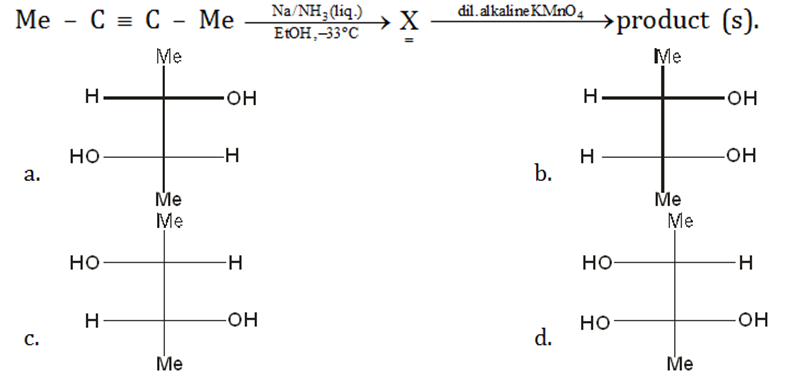Solution: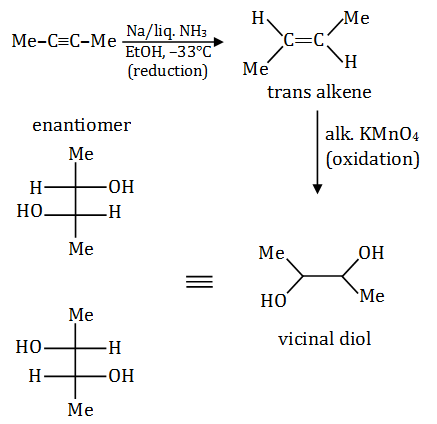In the above reaction, presences of Na in liquid NH3 alkynes reduce to give trans alkene. Alkaline KMnO4 gives vicinal diol as an oxidation product. These diols are enantiomers of each other.

Question 36. Which of the following give(s) a Meso compound as the main product?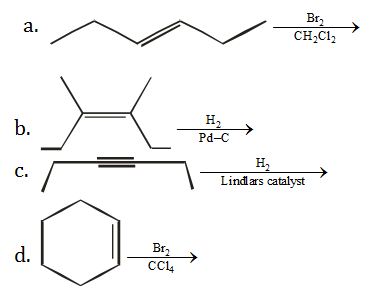Solution: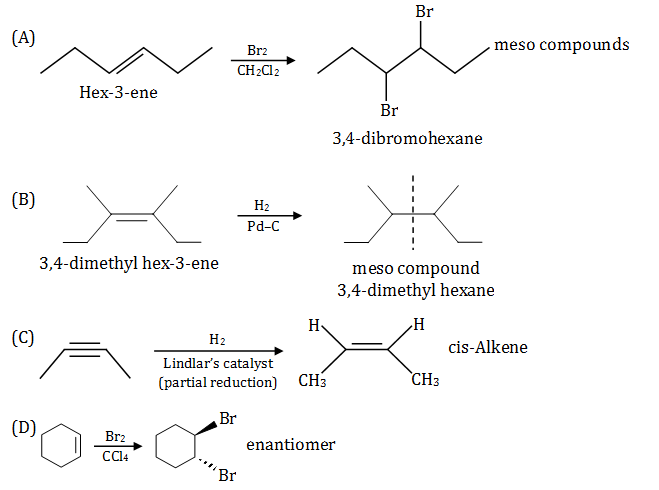Question 37. For spontaneous polymerization, which of the following is (are) correct?

1. a. ∆G is negative
2. b. ∆H is negative
3. c. ∆S positive
4. d. ∆S is negative

Solution:

The free energy change for any polymerization will be,

∆G = Gpolymer -Gmonomer

So, ∆G = ∆H – T∆S

∆T = (Hpolymer – Hmonomer) – T(Spolymer – Smonomer)

When the polymer has lower free energy than the initial monomer, polymerisation can occur spontaneously and the sign of ∆G is negative. ∆H is negative due to it's an exothermic process. Entropy change for polymerisation is also negative because polymers are orderly arranged.

Question 38. Which of the following statement(s) is/are incorrect:

1. a. A sink of SO2 pollutant is O3 in the atmosphere.
2. b. FGD is a process of removing NO2 from the atmosphere.
3. c. NO2 in fuel gases can be removed by alkaline scrubbing.
4. d. The catalyst used to convert CCl4 to CF4 by HF is SbF6.

Solution:

Fuel gas desulphurisation (FGD) process is used to remove sulphur from exhaust fuel gases. In this system, the sulphur removed from the fuel gas is converted into its elemental form or into sulphuric acid.

Alkaline scrubbing is used to remove SO2 from combustion exhaust gas.

Question 39. The total number of alkyl bromides (including stereoisomers) formed in the reaction

Me3 –C–CH =CH2+HBr -> will be

1. a. 1
2. b. 2
3. c. 3
4. d. No bromide forms

Solution:

For chemical reaction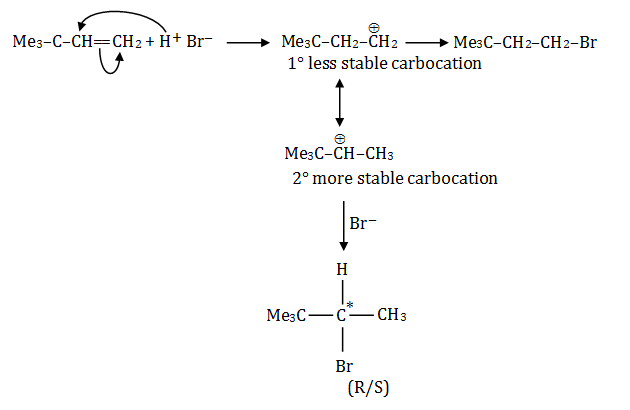The total number of stereoisomers possible for 4,4-dimethyl-pentene are three. As the molecule contain one chiral carbon atom.

Number of R and S isomers = 2n–1 = 21–1 = 2

So, total number of stereo isomers possible are = 2+1 = 3.

Question 40. How and why does the density of liquid water change on prolonged electrolysis?

1. a. Decreases, as the proportion of H2O increases
2. b. Remains unchanged
3. c. Increases as the proportion of D2O increases
4. d. Increases, as the volume decreases

Solution:

In electrolysis, water is decomposed in the presence of electricity, to produce hydrogen gas and oxygen gas.

The half reaction,

Reduction at cathode: 2H+(aq.)+2e -> H2(g)

Oxidation at anode: 2H2O(l) -> O2(g) + 4H+(aq.) + 4e

Over all reaction: 2H2O(l) -> 2H2(g)+O2(g)

On electrolysis volume of gases produced increases.

The relation between density and volume.

Density = mass/volume

As the volume increases density decreases, so as the proportion of water molecules increases density decreases.

### WBJEE 2020 Chemistry Question Paper with Solutions